Wu, X., Xu, J., and Hao, J. (2015). "Calculating elastic constants of binderless bamboo-wood sandwich composite," BioRes. 10(3), 4473-4484.

Abstract

Bending and shear stiffness, which are used as deformation resistance indexes, are very important mechanical properties of bamboo-wood sandwich composites. Thus, a new methodology to calculate the elastic constants of this type of material was proposed in this paper. First, the elastic constants of the composites were derived based on composite mechanics. In particular, the equivalent shear stiffness and modulus were determined by the energy method. Then, the three-point bending test and a revised three-point bending test were used to verify the accuracy of the theoretical model, which uses the properties of the skin and core layers as its input parameters. The model was subsequently evaluated. The results show that, generally, the predicted values were slightly smaller than the test results for the same bamboo-wood composite because of the strengthening of the wood veneer after hot-pressing.

Calculating Elastic Constants of Binderless Bamboo-Wood Sandwich Composite

Xinfeng Wu,a Jianying Xu,a,* and Jingxin Hao b

Bending and shear stiffness, which are used as deformation resistance indexes, are very important mechanical properties of bamboo-wood sandwich composites. Thus, a new methodology to calculate the elastic constants of this type of material was proposed in this paper. First, the elastic constants of the composites were derived based on composite mechanics. In particular, the equivalent shear stiffness and modulus were determined by the energy method. Then, the three-point bending test and a revised three-point bending test were used to verify the accuracy of the theoretical model, which uses the properties of the skin and core layers as its input parameters. The model was subsequently evaluated. The results show that, generally, the predicted values were slightly smaller than the test results for the same bamboo-wood composite because of the strengthening of the wood veneer after hot-pressing.

Keywords: Bamboo-wood sandwich composite; Elastic constants; Bending experiment; Mechanical properties

Contact information: a: College of Material Science and Engineering, Central South University of Forestry and Technology, Changsha, Hunan, China; b: College of Furniture and Art Design, Central South University of Forestry and Technology, Changsha, Hunan, China;

* Corresponding author: xjianying@hotmail.com

INTRODUCTION

It is well known that bamboo, which is planted across a wide range of Southern China, is plentiful. Bamboo, as an important renewable resource, is widely used in furniture production and building structures (Nogueira 2008). Its physical and mechanical characteristics, such as its morphology, availability, and low cost, make its use predominant, especially in the tropical and subtropical areas of Asia in which bamboo is used for food, shelter, tools, and other objects (Murad 2007). The difference in the dimensions and void in the core of bamboo mean that raw material utilization is very low and a lot of residue is produced; it is a challenge to reuse these wastes to protect the environment, improve revenues of forest zone farmers, and add value to a product.

Binderless gluing is a recent innovation in wooden composite production. In this type of gluing, artificial resin is not used as a binder; instead, sugar and acid are used as additives or self-bonding is achieved by steam or heat treatment (Okuda and Sato 2004; Luo et al. 2014). The mechanism of binderless gluing includes degradation of hemicelluloses and part of the cellulose fraction to produce sugars (Rowell et al. 2002), cross-linking between carbohydrate polymers and lignin (Suzuki et al. 1998), and an increase in cellulose crystallinity (Tanahashi 2002).

According to previous tests (Wu et al. 2015), the strength and stiffness of binderless bamboo particle board prevent its use in key architecture and furniture applications, so sandwich composite with binderless bamboo particle board reinforced with wood veneer was used to solve above problems. The main function of the reinforcing skin is to impart resistance to bending; the core is more resistant to shear force (Hao et al. 2014). The bending and shear deformation are carried by transverse loading, especially for small length-height ratios and soft cores. Therefore, bending and shear stiffness were used to characterize the deformation of the composites.

The earlier method to calculate bending and shear stiffness was proposed by Allen (1969), who carried out three-point bending test by changing span lengths and slope coefficient was plotted in linear graphs. Sometimes, the linear slope is close to the horizontal so that it is difficult to decide the coefficient. In addition, Howard (1962) proposed five-point bending test method to decide composite elastic constants, but the loading was very difficult to carry out. After that, a revised test method combining three-point bending test with five-point bending test was proposed by Li (1992). Compared to the normal three-point bending test, the transverse displacement is not only tested in the middle of the span, but is also tested in the end of the overhang; therefore the constants can be decided easily by derived equation. Nowadays, it is also used in many research areas.

The objective of this study was to develop a new methodology to calculate the elastic constants of such materials. Based on this method, the composite constants can be predicted directly by input of different lamina properties. First, the elastic constants of the composite were derived based on composite mechanics and the energy method. Then, the three-point bending test and a revised three-point bending test were used to verify the accuracy of the proposed theoretical model.

MATERIAL AND METHODS

Materials

Poplar veneer with thickness 2-mm was purchased from Xuzhou City in the Jiangsu Province. The moisture content of the veneer was maintained at about 11 to 12% via conditioning. It was cut to dimensions of 400 × 400 mm without knots or cracks. Bamboo chips (Phyllostachys pubescens) from processing residue were dried to 11 to 12% moisture content (Fig. 1) and the ratio of different dimensions and sizes is shown in Table 1. The natural additive, regarded as the material binder, includes substances of organic acids and surges.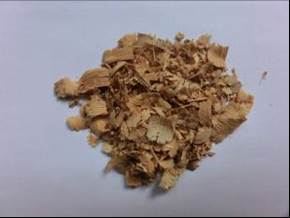Fig. 1. Sliced bamboo chips

Table 1. Different Dimension Ratios of Bamboo Chips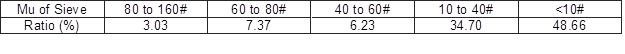The bamboo chips were weighed according to target core thicknesses of 9 and 12 mm and a target core density of 0.7 g/cm3 for each board. An aqueous solution of additives, at a 20% weight ratio on the chip weight, was added. The additive and chips were manually mixed and stirred in a plastic pot. Meanwhile, the back of the poplar veneer was sprayed with an aqueous solution of the additive at an application density of 180 g/m2. The sandwich construction with the veneer skin and bamboo chip core was assembled and manually pre-pressed. Next, it was pressed for 20 min at 170 °C and up to 4 MPa. Generally, the actual pressure applied to the panel was lower than 4 MPa because a thickness gage was used. After that, the bamboo-wood composite was cooled and conditioned for 2 d.

Theoretical Methods

Derivation of equivalent bending stiffness and modulus

As shown in Fig. 2, the sandwich composite includes three lamina, denoted i = 1, 2, and 3. The x-coordinate is the longitudinal direction of the sandwich beam and the z-coordinate is the transverse direction.Fig. 2. Construction notification of sandwich composite

According to composite mechanics, the bending stiffness of a sandwich beam can be expressed as in Eq. 1,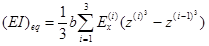(1)

where (EI)eqbEx(i), and z(i) denote the equivalent bending stiffness of the sandwich beam, the beam width, the ith-layer longitudinal modulus, and the z-coordinate of the interface between (i+1)-th layer and the i-th layer, respectively.

If a homogeneous, single-layer beam is used instead of a sandwich composite with the same cross-section (Eq. 2), then,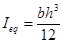(2)

where Ieq and h denote the equivalent cross-sectional moment of inertia and the height of the sandwich beam, respectively.

Therefore, the equivalent bending modulus is (Eq. 3),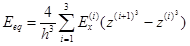(3)

where Eeq denotes the equivalent bending modulus of the sandwich beam.

Derivation of equivalent shear stiffness and modulus

The shear strain energy density at any position of a sandwich beam is (Eq. 4),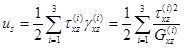(4)

where us denotes the shear strain energy density of the sandwich beam and τxz(i),γxz(i), and Gxz(i)denote the ith -layer shear stress, shear strain, and shear modulus, respectively.

Generally, the lamina is considered symmetrical around the centroid of the cross-section to balance deformation, which yields (Eq. 5),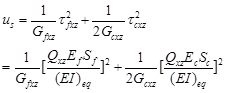(5)

where τfxzτfxzGfxzGcxzEfEc, and Qxz denote the skin stress, core stress, skin shear modulus, core shear modulus, skin longitudinal modulus, core longitudinal modulus, and transverse shear force, respectively. Sf and Sc can be represented as (Eq. 6),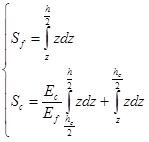(6)

As for a rectangular cross section, Sf and Sc can be expressed in another way (Eq. 7),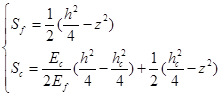(7)

where hc denotes the core thickness of the sandwich beam.

Therefore, the total shear strain energy of sandwich beam is determined as (Eq. 8),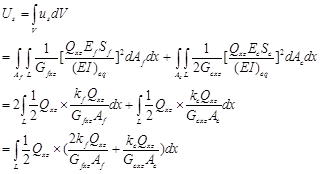(8)

where Af and Ac denote the cross-sectional area of the skin and core layers, respectively. The quantities kf and sc are expressed as (Eq. 9),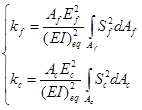(9)

Assuming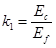and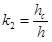for a rectangular cross section (Eq. 10),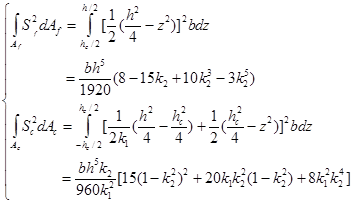(10)

Then kf and kc can be expressed as (Eq. 11),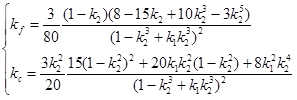(11)

If the total shear strain energy of the sandwich beam is calculated using the average strain, it can be expressed in another way (Eq. 12),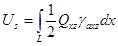(12)

where γaxz denotes the average shear strain of the cross-section.

Integrating Eq. 12 in Eq. 8 yields (Eq. 13),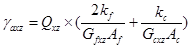(13)

Thus, γaxz is defined as (Eq. 14),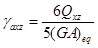(14)

where (GA)eq denotes the equivalent shear stiffness, and 6/5 is the average shear strain coefficient of a rectangular cross-section for a homogeneous, single-layer beam.

Then (Eq. 15),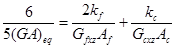(15)

Therefore, the equivalent shear stiffness is (Eq. 16),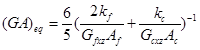(16)

Equation16 can also be written generally as (Eq. 17),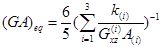(17)

If a homogeneous, single-layer beam is used instead of a sandwich composite with the same cross-section, then the equivalent shear modulus is (Eq. 18),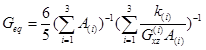(18)

where Geq denotes the equivalent shear modulus.

Experimental Methods

Test Methods

According to GB/T 17657-2013, the equivalent bending modulus is (Eq. 19),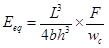(19)

where FL, and wc denote the transverse loading, span distance, and transverse displacement in the middle of the span, respectively. Generally, the quantity F/wc is used instead of F/wc in Eq. 19 to calculate the modulus during the linear stage of testing.

Using Eq. 2, the equivalent bending stiffness can be derived (Eq. 20),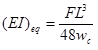(20)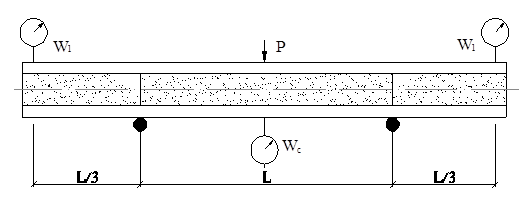Fig. 3. Theory of revised three-point bending test on sandwich beam

Due to the inability to calculate the shear stiffness and modulus using Eqs. 19 and 20, a revised three-point bending test method was proposed in Fig. 3 (Li et al. 1992). The equivalent bending stiffness and shear stiffness are then (Eq. 21),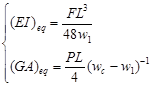(21)

where w1 denotes the transverse displacement in the end of the overhang.

Finally, the equivalent bending modulus and shear modulus are derived as (Eq. 22),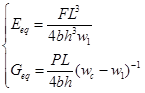(22)

Table 2. Equation Summary to Calculate Composite Constants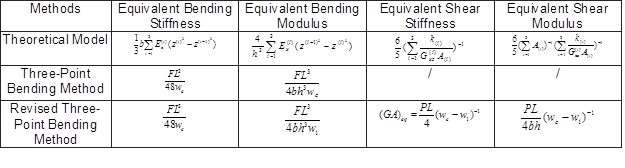The equations used to calculate the composite’s constants are summarized in Table 2. Based on these theories, the bamboo-wood sandwich composite was sawn into test specimens with dimensions of 350 × 50 mm. All tests were carried out using a universal mechanical testing machine (KHQ-002) manufactured by Suzhou Jianhao Instrument Company, China. Detailed test parameters are shown in Table 3. For the revised three-point bending test, the loading method is shown in Fig. 4. The transverse displacement in the middle of the span can be recorded automatically, but the displacement of the overhang should be determined using a micrometer gauge.

Table 3. Parameters of Bending Test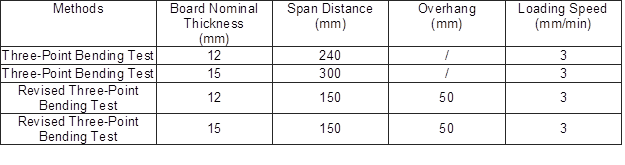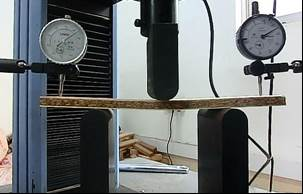Lamina Property Test

Due to the thin size and softness of the poplar veneer, it was impossible to test the bending modulus and shear modulus of the lamina using either the revised three-point bending test or the reference standard method. A 20-mm poplar beam was used instead of veneer to test the elastic constants of poplar. The mechanical properties are shown in Table 4. Considering that the veneer was compressed during the gluing process, the poplar beam was also compressed under the same conditions and the corresponding modulus was tested.

When testing the core properties, the skin of the wood veneer should be removed from the sandwich composite via sanding. The core layer can then be tested using the revised three-point bending test. The determined properties are shown in Table 5.

Table 4. Property and Modulus of Poplar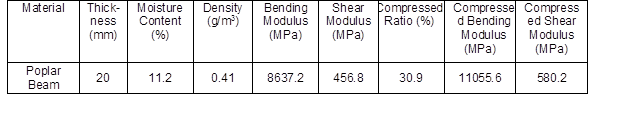Table 5. Property and Modulus of Core Layer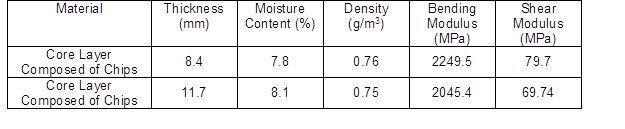RESULTS AND DISCUSSION

Table 6. Comparison between Theoretical Model and Test Values of Elastic Constants of Bamboo-wood Composites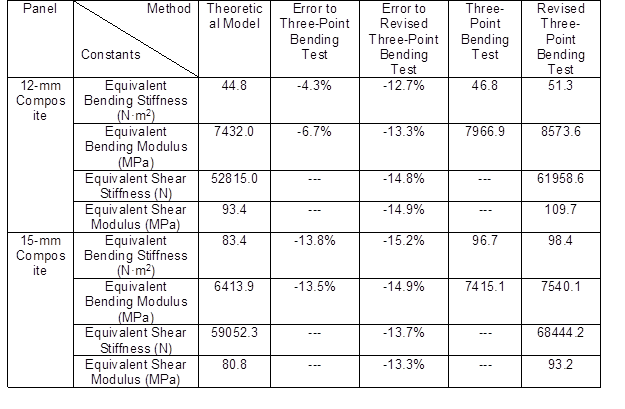Comparisons between the theoretical model and test values of the elastic constants of the bamboo-wood composite are shown in Table 6. Generally, the average error between the different calculation methods was about 10 to 16% and the predicted values were slightly smaller than test results indicated for the same construction of the bamboo-wood composite. One possible reason is that the skin bending stiffness was strengthened after hot-pressing. As shown in Fig. 5, the additive permeated 0.8 to 1 mm into the veneer; thus, a gluing layer was formed to improve the stiffness and mechanical properties of the outer layer.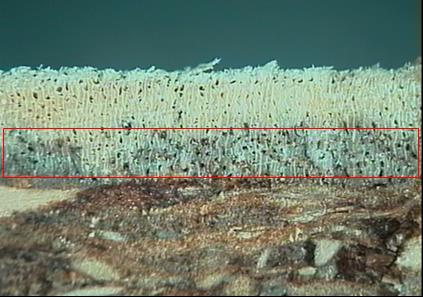Fig. 5. The gluing interface between the veneer and the core layer (100× magnification)

Actually, the modulus distribution varies along cross section of poplar skin due to property change caused by veneer’s production process, compression, and additive permeation at glue surface. But only the average bending modulus without additive permeation is considered as input parameters of theoretical model in this paper, which is a limitation of this method and will influence final prediction accuracy.

Compared to revised three-point bending test method (Li), of which results are sensitive to displacement measurement, composite constants can be decided easily from input of every lamina properties in the theoretical model. Therefore, the final test can be cancelled for the whole composite, which can save a lot time and costs in the application area.

CONCLUSIONS

1. The elastic constants of a composite were derived based on composite mechanics. Specifically, the equivalent shear stiffness and modulus were determined using an energy method.
2. The three-point bending test and a revised three-point bending test were used to verify the accuracy of the proposed theoretical model.
3. Generally, the predicted values were slightly smaller than the test results for the same bamboo-wood composites because of strengthening of the wood veneer after hot-pressing.

ACKNOWLEDGMENTS

This project was supported by the National 948 Foundation (Introduced Manufacture Process and Technique of Environment-Friendly, Binderless Particle Board:2011-4-22) and the Scientific Research Foundation of the Graduate School of the Hunan Province (Study on Mechanical Properties of Environment-Friendly Bamboo-Strip Sandwich Composite for Furniture: CX2012B315).

REFERENCES CITED

Allen, H. G. (1969). Analysis and Design of Structural Sandwich Panels, Pergamon Press, Oxford.

Hao, J. X., Wu, X. F., and Liu, W. J. (2014). “Modeling and verification of sandwich beam with wooden skin and honey-comb core subjected to transverse loading,” Scientia Silvae Sinicae 50(7), 128-137. DOI: 10.11707/j.1001-7488.20140718.

Howard, H. B. (1962). “The five-point shear stiffness test,” J. Roy. Aeron. Soc. (66), 621.

GB/T 17657 (2013). “Test methods of evaluating the properties of wood-based panels and surface decorated wood-based panels,” Standardization Administration of the People’s Republic of China, Beijing, China.

Li, J. J., and Yang, G. C. (1992). “The progression and determination of elastic constants of honeycomb sandwich panels,” Fiber Reinforced Plastics/Composites (5), 6-13.

Luo, H., Yue, L., Wang, N. W., Zhang, H. Y., and Lu. X. N. (2014). “Manufacture of binderless fiberboard made from bamboo processing residues by steam explosion pretreatment,” Wood Research59(5), 861-870.

Murad, J. R. L. (2007). “As propriedades fisicas, mecanicas e mesoestrutural do bambu guadua weberbaueri do acre,” M.S. thesis, Pontificia Universidade Catolica do Rio de Janeiro, Rio de Janeiro, Brazil.

Nogueira, C. L. (2008). Painel de bambu laminado colado estrutural,” M.S. thesis Escola Superior de Agricultura Luiz de Queiroz da Universidade de Sao Paulo, Piracicaba, Brazil.

Okuda, N., and Sato, M. (2004). “Manufacture and mechanical properties of binderless boards from kenaf core,” J. Wood. Sci. 50(1), 53-61.

Rowell, R. M., Lange, S., McSweeny, J., and Davis, M. (2002). “Modification of wood fiber using steam,” in: Proceedings of the 6th Pacific Rim Bio-Based Composites Symposium & Workshop on the Chemical Modification of Cellulosics, Portland, Oregon, USA, 2, 606-615.

Suzuki, S., Shintani, H., Park, S. Y., Saito, K., Laemsak, N., Okuma, M., and Ilyama, K. (1998). “Preparation of binderless boards from steam exploded pulps of oil palm (Elaeis guineensis Jacq.) fronds and structural characteristics of lignin and wall polysaccharides in steam-exploded pulps to be discussed for self-bindings,” Holzforschung 52(4), 417-426.

Tanahashi, M. (2002). “Assembled wood production from small logs without adhesives by the compressive molding process,” in: Proceedings of the 5th Pacific Rim Bio-based Composites Symposium, Canberra, Australia.

Wu, X. F., Xu, J. Y., Hao, J. X., Liao, R., and Zhong, Z. (2015). “Properties of binderless bamboo particleboard,” Journal of Functional Materials 46(9), 09092-09095.

Article submitted: March 25, 2015; Peer review completed: May 22, 2015; Revised version received: May 21, 2015; Accepted: May 22, 2015; Published: June 1, 2015.

DOI: 10.15376/biores.10.3.4473-4484